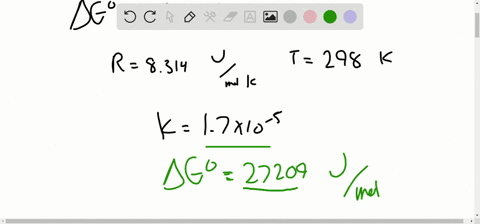Enroll in one of our FREE online STEM summer camps. Space is limited so join now!View Summer CoursesCheck back soon!

## Discussion

You must be signed in to discuss.

## Video Transcript

were given this reaction, and we want to determine the partial pressure at equilibrium for carbon dioxide gas, a 25 degree Celsius. We can begin by rating out the expression for the equilibrium constant K p. We know that when we're writing out that expression, that solid species do not participate in that that expression that we right out we have a curious species, then we're looking for a value of K C, since we have concentration values. But the only non solid that we have in this equation is this carbon dioxide gas, and so we use KP. Since gas is, gas concentrations are represented with partial pressure values. And so when we write out the KP expression, we take the partial pressure of each gas on the product side of the reaction, and we raise it to the power of its tricky metric coefficient. And then we multiply that quantity by each gas in the products and then divide that same those same values for all the gases on the reactions. But since we just have one single gas on the product side CO two, that means that the equilibrium expression KP is just equal to the partial pressure of CO two since has a stroke geometrically efficient of one ensue. We know that there is an equation, then to find the equilibrium constant, using the changing Gibbs free energy of the reaction. So if we plug in values to sell for that that value for the equilibrium constant, we know that that will also equal the partial pressure of CO two at those conditions. So next we need to solve for the change in Gibbs free energy of the reaction. We use this equation on the bottom to help us do that. The first find the total change and gives free energy of formation at standard conditions of the products and then subtract that seemed value from of the for the reactions. You can break up the turns into the products in the react mints and starting with the product side, we see that we have one mole of CEO in one mole of CO two, so one mole of CEO on one mole of CO two or each multiplied by their values. For Delta G information, it's danger conditions which we can look up in the appendix so that units of moles cancel out to yield total units of energy for the change in Gibbs free energy of the products. And now for the reactions, we see that we have one mole of C A c 03 And when we multiply that with its delta G information at standard conditions value, you get the total Gibbs free energy change of the reactions. Then we take the total delta G of the products and subtract the total delta G of the reactant. We do that, we get this final answer for Delta G of reaction. And now we can plug that in for this variable in the equation that we are using the cell for the partial pressure of co two. So KP equals E to the power of negative Delta G, which is what we just saw for 130 0.9. And we need to convert this into jewels to the weekend, eventually cancel out the units of the gas Constant are so we should multiply this by 1000. Get it in jewels per mole. Then we divide this by are the constant eight point 314 Jules or more times Kelvin and then multiply that by the temperature. If we're told is 298 Teldyn, and now we just plug that all in. And we knew again from this relationship that that equilibrium constant will be equal to the partial pressure of CO two, which is what we're ultimately trying to find. So we can see that the partial pressure of CO two at equilibrium at 25 degrees Celsius for this system is equal to about 1.13 times and through the negative 23rd power in atmospheres for the partial pressure units.